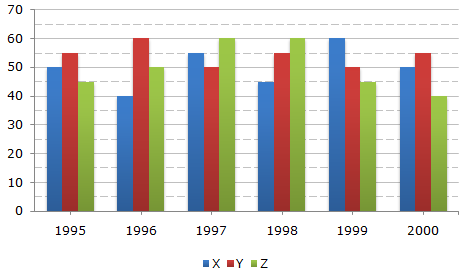# Data Interpretation - Bar Charts

Directions to Solve

A soft drink company prepares drinks of three different flavours - X, Y and Z. The production of three flavours over a period of six years has been expressed in the bar graph provided below.

Production of Three Different Flavours X, Y and Z by a Company over the years (in lakh bottles)1.
The total production of flovour Z in 1997 and 1998 is what percentage of the total production of flavour X in 1995 and 1996?
96.67%
102.25%
115.57%
133.33%
Explanation:

Required percentage
 =(60 + 60) x 100% (50 + 40)
 =120 x 100% 90
= 133.33%.

2.
For which flavour was the average annual production maximum in the given period?
X only
Y only
Z only
X and Y
Explanation:

Average annual productions over the given period for various flavours are:

 For Flavour X =1 x (50 + 40 + 55 + 45 + 60 + 50)= 50 lakh bottles. 6

 For Flavour Y =1 x (55 + 60 + 50 + 55 + 50 + 55)= 54.17 lakh bottles. 6

 For Flavour Z =1 x (45 + 50 + 60 + 60 + 45 + 40)= 50 lakh bottles. 6Maximum average production is for Flavour Y.

3.
What is the difference between the average production of flavour X in 1995, 1996 and 1997 and the average production of flavour Y in 1998, 1999 and 2000?
50,000 bottles
80,000 bottles
2,40,000 bottles
5,00,000 bottles
Explanation:

Average production of flavour X in 1995, 1996 and 1997

 =1 x (50 + 40 + 55)3

 =145lakh bottles. 3

Average production of flavour Y in 1998, 1999 and 2000

 =1 x (55 + 50 + 55)3

 =160lakh bottles. 3Difference
 =160 - 1453 3
 = 15 3
= 5 lakh bottles
= 5,00,000 bottles.

4.
What was the approximate decline in the production of flavour Z in 2000 as compared to the production in 1998?
50%
42%
33%
25%
Explanation:

Percentage decline in the production of flavour Z in 2000 as compared to the production in 1998

 =(60 - 40) x 100% 60

 =20 x 100% 60

= 33.33%33%.

5.
For which of the following years the percentage of rise/fall in production from the previous year is the maximum for the flavour Y?
1996
1997
1998
1999
Explanation:

The percentage rise/fall in production from the previous year for flavour Y during various years are:

 In 1996 =(60 - 55) x 100% = 9.09% (increase) 55

 In 1997 =(60 - 50) x 100% = 16.67% (decrease) 60

 In 1998 =(55 - 50) x 100% = 10% (increase) 55

 In 1999 =(55 - 50) x 100% = 9.09% (decrease) 55

 In 2000 =(55 - 50) x 100% = 10% (increase) 50Maximum change is decrease of 16.67% during 1997.